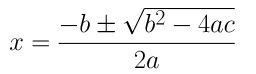Guru

# Find the nature of the roots of the following quadratic equations. If the real roots exist, find them;(iii) 2×2 – 6x + 3 = 0 Q.1(3)

• 0

What are the best way for solving ncert 10th solution, of quadratic equation exercise 4.4. How i solve this question in easy way Find the nature of the roots of the following quadratic equations. If the real roots exist, find them;(iii) 2×2 – 6x + 3 = 0

Share

1. 2x2 – 6x + 3 = 0

Comparing the equation with ax2 + bx c = 0, we get

a = 2, b = -6, c = 3

As we know, Discriminant = b2 – 4ac

= (-6)2 – 4 (2) (3)

= 36 – 24 = 12

As b2 – 4ac > 0,

Therefore, there are distinct real roots exist for this equation, 2x2 – 6x + 3 = 0.= (-(-6) ± √(-62-4(2)(3)) )/ 2(2)

= (6±2√3 )/4

= (3±√3)/2

Therefore the roots for the given equation are (3+√3)/2 and (3-√3)/2

• -1